# Vietoris homology

An (ordered)-dimensional-simplex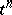of a subsetof a metric spaceis defined as an ordered subset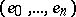insubject to the condition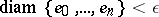. The-chains ofare then defined for a given coefficient groupas formal finite linear combinationsof-simplices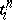with coefficients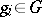. The boundary of an-simplex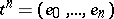is defined as follows:; this is an-chain. By linearity, the boundary of any-chain is defined and-cycles are defined as-chains with zero boundary. An-chainof a set is-homologous to zero in(the notation is) iffor a certain-chain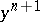in.
A true cycle of a setis a sequence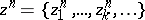in which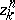is an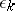-cycle inand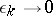(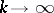). The true cycles form a group,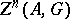. A true cycleis homologous to zero inif for anythere exists ansuch that allforare-homologous to zero in. One denotes by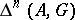the quotient group of the groupby the subgroup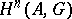of cycles that are homologous to zero.
A cycleis called convergent if for anythere exists ansuch that any two cycles,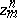are mutually-homologous inif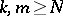. The group of convergent cycles is denoted by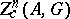. Let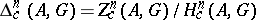be the corresponding quotient group.
A cyclehas compact support if there exists a compact setsuch that all the vertices of all simplices of all cycleslie in. One similarly modifies the concept of a cycle being homologous to zero by requiring the presence of a compact set on which all the homology-realizing chains lie; convergent cycles with compact support can thus be defined. By denoting with a subscriptthe transition to cycles and homology with compact support, one obtains the groups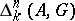and. The latter group is known as the Vietoris homology group. If the polyhedron is finite, the Vietoris homology groups coincide with the standard homology groups.
Relative homology groups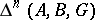,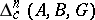,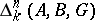,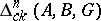modulo a subset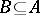are also defined. An-cycle of the setmodulois any-chaininfor which the chain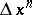lies in. In a similar manner, an-cyclemodulois-homologous moduloto zero inif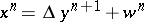, whereand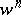are-chains in, while the chainlies in.